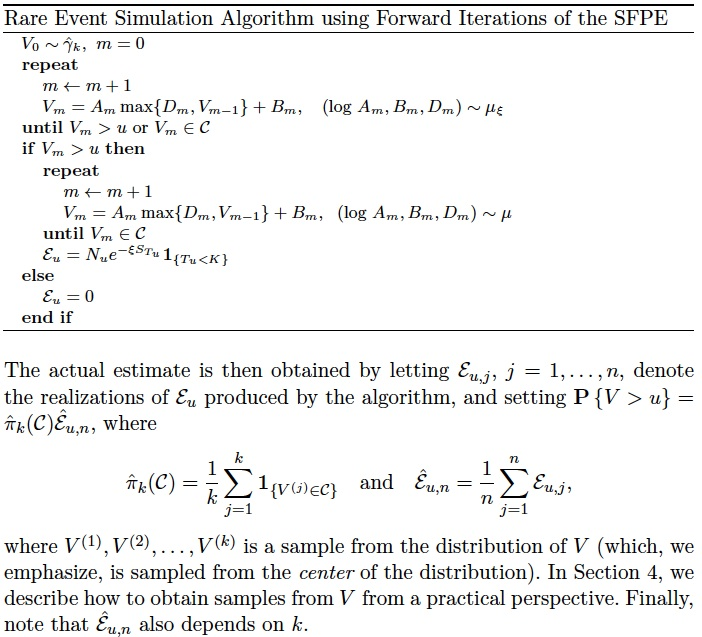## RESSFPE :: Rare Event Simulation for Processes Generated via SFPE

RESSFPE implements the importance sampling algorithm for estimating rare event probabilies arising from stochastic fixed point equations, as described in Collamore, Diao, and Vidyashankar ( Annals of Applied Probability, 2013).

### General Information

The program is written in C language. Executable file for Linux/Mac platform is available in the download section below.

### Usage

On Linux systems:

 res_sfpe_linux 

On Mac systems:

 res_sfpe_mac 

### Input

The program implements the importance sampling algorithm for estimating the tail probabilities for three types of processes: (1) ruin problem with stochastic investments; (2) ARCH(1); and (3) GARCH(1,1).

1. In the ruin problem with stochastic investment, we consider the following SFPE

2. The ARCH(1) process is modeled by by the recurrence equation

3. The GARCH(1,1) process is modeled by by the recurrence equation

Model Parameters
Ruin problem with investment
ARCH(1)
GARCH(1,1)

### AlgorithmTo estimate the tail probabily P(V>u), besides the model parameters in the above input section, one also needs to specify: (1) k; (2) n; and (3) u.

### Output

Computational results include the adjustment coefficient such that , the tail probability P(V>u) and its 95% confidence limits, and the relative error.

### Example» res_sfpe_linux

» res_sfpe_mac